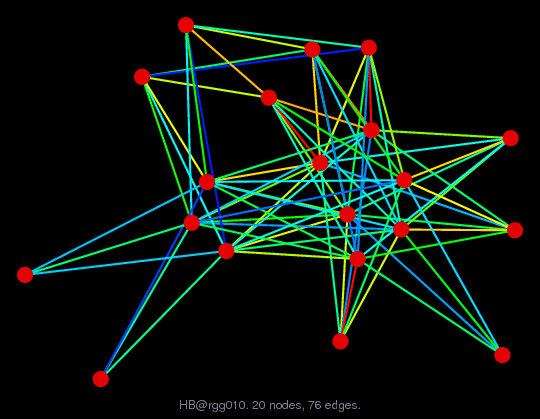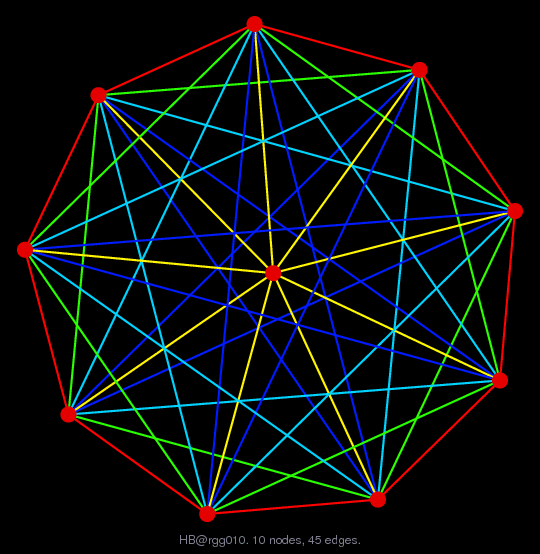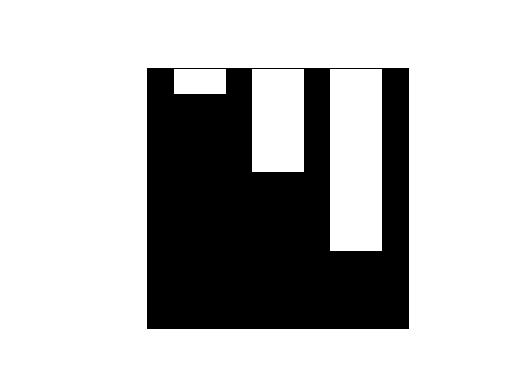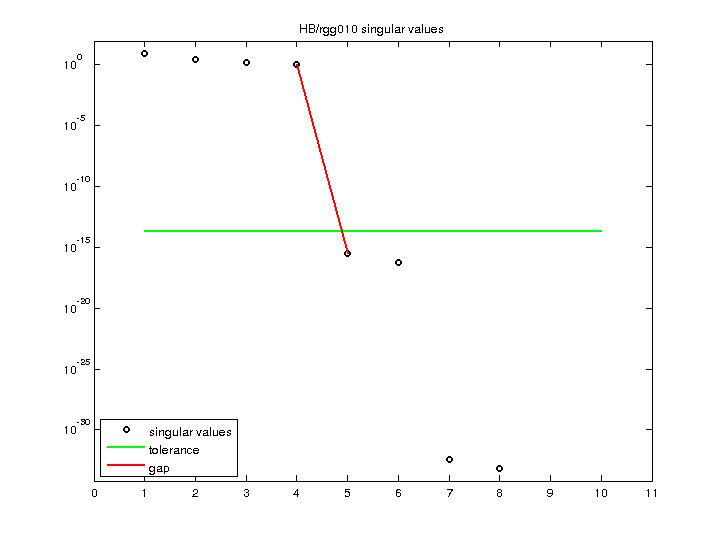Matrix: HB/rgg010

Description: S EXAMPLE TO DEMONSTRATE THE CHIMNEY EFFECT IN P4 - BY R.G. GRIMES(bipartite graph drawing) (graph drawing of A+A')• Matrix group: HB
• download as a MATLAB mat-file, file size: 528 bytes. Use UFget(238) or UFget('HB/rgg010') in MATLAB.

 Matrix properties number of rows 10 number of columns 10 nonzeros 76 structural full rank? yes structural rank 10 # of blocks from dmperm 1 # strongly connected comp. 1 explicit zero entries 0 nonzero pattern symmetry 64% numeric value symmetry 64% type binary structure unsymmetric Cholesky candidate? no positive definite? no

 author J. Lewis editor I. Duff, R. Grimes, J. Lewis date 1983 kind counter-example problem 2D/3D problem? no

 Ordering statistics: result nnz(chol(P*(A+A'+s*I)*P')) with AMD 55 Cholesky flop count 3.8e+02 nnz(L+U), no partial pivoting, with AMD 100 nnz(V) for QR, upper bound nnz(L) for LU, with COLAMD 55 nnz(R) for QR, upper bound nnz(U) for LU, with COLAMD 55

 SVD-based statistics: norm(A) 8.19707 min(svd(A)) 0 cond(A) Inf rank(A) 4 sprank(A)-rank(A) 6 null space dimension 6 full numerical rank? no singular value gap 3.34702e+15

 singular values (MAT file): click here SVD method used: s = svd (full (A)) status: ok